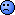## tmax (DR & multiphasic) [Regulatives / Guidelines]

Dear all,

another goody. Lines 811–814

[…] A formal statistical evaluation of tmax is not required. However, there should be no appa­rent difference in median tmax and its range between test and reference product.

That’s truly clever. OK, we all learned in nursery school that nonparametric statistics are poisonous. (Concerning risks and adverse effects read the SmPC and ask your physician or pharmacist.)1
The IR GL states:

However, […] there should be no apparent difference in median tmax and its variability between test and reference product.

Do you get the subtle distinction? I was always wondering what the variability of the median might be in the EMA’s opinion (without applying nonparametric statistics this generally ended up in more or less eloquent). Now I’m enlightened. It is the range! Splendid idea! A statistical parameter with a breakdown point2 of zero. Not even a dyed-in-the-wool nonparametrician on the maximum allowed dose of Schützenafil® would ever compare data based on their ranges. Maybe the IQR performs better?3

2. The breakdown point of an estimator is defined as the number of incorrect values which will not lead to a biased result.
• The arithmetic mean has a breakdown point of 1: Imagine an (even infinitely large) set of identical values (say 1). Now change a single original value to $$\small{\infty}$$. The arithmetic mean of the new set is $$\small{\infty}$$.
$$x_1=1,x_2=1,\ldots, x_{i=n}=1\rightarrow \bar{x}=\frac{1}{n}\sum_{i=1}^{i=n}x_i=1$$
$$x_1=1,x_2=1,\ldots, x_{i=n}=\infty\rightarrow \bar{x}=\frac{1}{n}\sum_{i=1}^{i=n}x_i=\infty \; \small{\square}$$
• The median has a breakdown point of 50%. You would need to change 50% of values to ∞ in order to contaminate the median. Therefore, the median is a robust estimator of location.
• Unfortunately the range performs just as bad as the arithmetic mean. Only two values of the entire data set (min & max) are used. Everything else is ignored. Imagine two (if you like very large) ranked sets:
  R  {1, …, 1, 2} median 1, range 1   T1 {1, …, 1, 3} median 1, range 2 → apparent differenceOr more weird:
  T2 {1, …, 1, 1} median 1, range 0 → superior?
3. Duno. Try:
t   <- c(0.5, 0.67, 0.83, 1, 1.33, 1.67, 2) p   <- c(0.025, 0.1, 0.15, 0.45, 0.15, 0.1, 0.025) n   <- 24 set.seed(1234567) R   <- sample(t, size=n, replace=TRUE, prob=p) T   <- sample(t, size=n, replace=TRUE, prob=p) med <- c(median(R), median(T)) rng <- c(range(R), range(T)) iqr <- c(IQR(R), IQR(T)) f   <- "%s%3.2f%s%3.2f%s%+3.2f%s" op  <- par(no.readonly = TRUE) par(family="mono") boxplot(R, T, range = 0, names = c("R", "T"), ylim = c(0, max(t)), las = 1) legend("bottomright", box.lwd = 0, box.col = "white", legend = paste(   sprintf(f, " Medians: R=", med, ", T=", med,              " (\u2206=", med-med, ")"), "\n",   sprintf(f, "Ranges : R=", rng, ", T=", rng,              " (\u2206=", rng-rng, ")"), "\n",   sprintf(f, "IQRs   : R=", iqr, ", T=", iqr,              " (\u2206=", iqr-iqr, ")"), "\n"), cex = 0.9,   title = "Statistics of Tmax") points(x = jitter(rep(1, n), 10), R) points(x = jitter(rep(2, n), 5), T) box() par <- op
BTW, what’s “no apparent difference”?

Dif-tor heh smusma 🖖🏼 Довге життя Україна!Helmut SchützThe quality of responses received is directly proportional to the quality of the question asked. 🚮
Science QuotesIng. Helmut Schütz